# LEN Function

Returns the length of a given text string

## What is the LEN Function?

The LEN Function is categorized under Excel TEXT functions. The function will return the length of a given text string.

In financial analysis, the LEN function can be useful if we wish to get the length of a given text string as the number of characters. LEN will also count characters in numbers, but number formatting is not included.

### Formula

=LEN(text)

The LEN function uses only one argument:

1. Text (required argument) – This is the text for which we wish to calculate the length. We can provide the text argument for the function:

• Directly
• As a string returned from another formula
• As a reference to a cell containing a string

### How to use the LEN Function in Excel?

As a worksheet function, LEN can be entered as part of a formula in a cell of a worksheet. To understand the uses of the function, let us consider an example:

#### Example 1

Let’s see how we can test a few conditions. Suppose we are given the following values: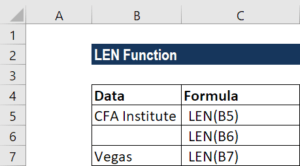We will get the results below: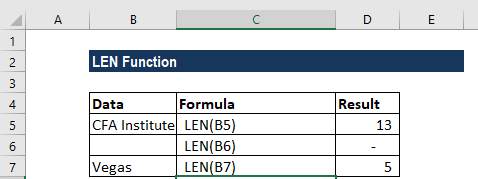In the examples above:

1. Spaces are counted as characters. Hence, the function returned 13 for the first argument.
2. The length of the empty string is 0.

#### Example 2

Let us now use the LEN function to remove characters from the data below: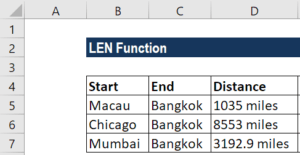We wish to remove miles from the Distance column. For removing the last n characters from a text string, we can use a formula based on the LEFT and LEN functions. We can use a formula to strip the last 5 characters of a value, starting on the left. The formula to use will be =VALUE(LEFT(D5
,LEN(D5)-6)).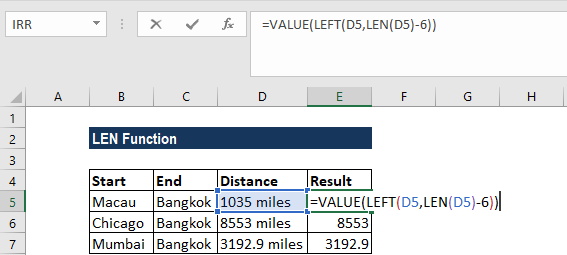We will get the result below: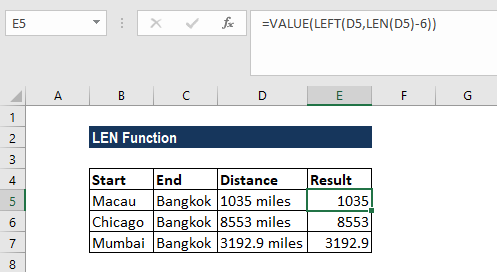In the formula above, the LEFT function extracted characters from the left side of a value as we specified. We then used the LEN function to calculate the total length of each value. For example, the length of 1035 miles is 9.

To get the number of characters to extract, we subtracted 6, which is the length of ” miles” (including the space character). The result is 4, which is fed to LEFT as the number of characters to extract. LEFT then returns the text “1035” as a text value.

As we wanted a numeric value and not a text value, we ran the text through the VALUE function, which converted numbers in text format to plain numbers.

Thanks for reading CFI’s guide to important Excel functions! By taking the time to learn and master these functions, you’ll significantly speed up your financial analysis. To learn more, check out these additional CFI resources:

• Excel Functions for Finance